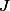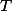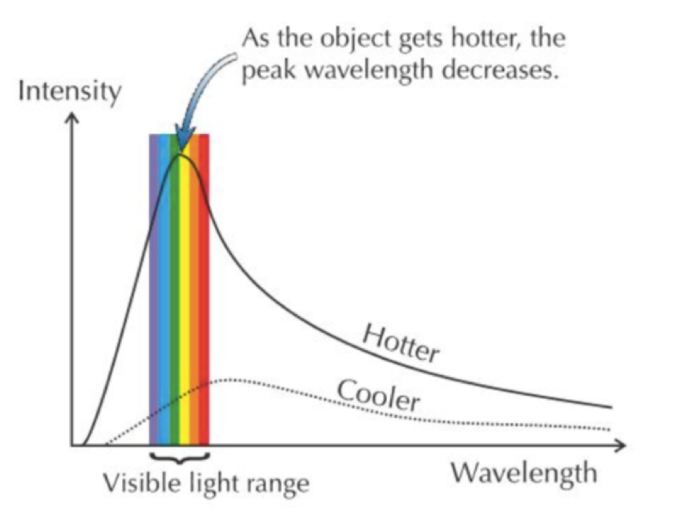# Stefan Boltzmann Law

## Stefan Boltzmann Law - Definition and Equation

Josef Stefan was an Austrian physicist. In 1879, he formulated a law about the radiant energy of a blackbody. According to the law, a theoretical object that absorbs all the radiation that falls on it which is proportional to the fourth power of its temperature. His law was one of the first important steps toward the understanding of the blackbody radiation, from which raised the quantum idea of radiation.

Stefan rose from a lecturer in the subject of mathematical physics in 1858 to a professor Ordinarius of physics in 1863 and to the director the Physical Institute in 1866, at the University of Vienna. Five years later he derived his law empirically. The same was derived theoretically by Ludwig Bolzmann of Austria and hence became known as the Stefan-Boltzmann Law.

Blackbody Radiation

A theoretical object that can be perfectly efficient at absorbing and emitting radiation is known as a blackbody, and it therefore emits what's known as blackbody radiation.

A blackbody is black at room temperature, hence the name. However, at higher temperatures, it can actually glow at visible wavelengths. Therefore, you should be aware that astronomers and physicists use the term 'blackbody' to also refer to objects that glow.

Naturally, we may tend to think that blackbody radiation is given off only by hot objects like that hot metal rod or an extremely hot star. Not so. The radiation that is emitted by a heated body is termed as Blackbody radiation. But 'heated' is a relative term. What I mean is, it's heated when compared to absolute zero - 0 Kelvin, -273.2 Celsius or -459.7 Fahrenheit.

Knowing this, it won't surprise you to learn that blackbody radiation is emitted by everything from stars and light bulbs to ice cubes. That's because at temperatures above absolute zero, particles in an object still have thermal energy and thus radiate photons. An ice cube may be as cool as a cucumber but compared to absolute zero, it's as agitated and hot as you are when your computer freezes or car doesn't start.

Why Does Hotter = Brighter?

Now we need to learn how the temperature and the color of the glow of an object interact. Surely you've seen how as metal is heated more and more, it actually changes the color of light it emits from red, to orange, to yellow, as it gets hotter. Now you'll learn why it changes color and gets brighter as it gets hotter, not just why it glows.

Firstly, the hotter an object is, the more blackbody radiation is emitted by it. Why? Well, the madder you are, the more agitated you are, and the more steam you have to blow off. The hotter an object, the more collisions there are, the more violent those collisions, and the more radiation is emitted. This is why as things get hotter, they get brighter.

Stefan-Boltzmann Law:

The Stephan-Boltzmann Law describes the power radiated a body that absorbs all radiation that falls on its surface in terms on its temperature. The radiation energy per unit time from a black body is proportional to the fourth power of the absolute temperature and can be expressed as the following formula.

Radiate energy = (Stefan-Boltzmann constant) × (Temperature)4

The equation is: J = σ T4 J m-2 s-1- Energy radiated per unit area by a blackbody per unit time [Units: J m-2 s-1]- Stefan-Boltzmann constant [Value: 5.67 × 10-8 J s-1 m-2 K-4]- Absolute temperature [Units: K]

A body that does not absorb all the radiations that are incident on it are known as a grey body which emits less total energy than a blackbody and is characterized by an emissivity, є<1.

J = єσ T4

The radiant emittance J has the dimensions of energy flux (energy per time per area), and the SI units of measurement are joules per second per square meter, or equivalently, watts per square meter. Kelvin is the SI unit for absolute temperature T. є is the emissivity of the grey body; if it is a perfect blackbody, є =1.

To find the total radiated power from an object, multiply the formula with its surface area A.

P = J × A = Aєσ T4

Radiation energy/Power can be represented as following:

Radiate energy = (Emissivity) × (Stefan-Boltzmann constant) × (Temperature)4 × (Area)

The equation is: P = є σ T4 A Watts
P: Radiate energy
σ: The Stefan-Boltzmann Constant
T: Absolute temperature in Kelvin
є: Emissivity of the material
A: Area of the emitting body

Pictorial representation of Stefan-Boltzmann law:

Check out the graphs below representing blackbody curves to see this concept represented visually. Note that these graphs have a y-axis indicating intensity and an x-axis representing wavelength.

You see larger areas under curves representing hotter objects, where the area under the curve is proportional to the total energy emitted. So, a hotter object emits more radiation (including visible light). This concept refers to the Stefan-Boltzmann Law, which says, in simple terms, that the total energy radiated from a blackbody is proportional to the fourth power of its temperature.What is Absolute Temperature?

The absolute temperature of an object is the temperature on a scale, where 0 is taken as absolute zero. This is also known as thermodynamic temperature; absolute temperature scales are Kelvin, degree units Celsius and Rankine degree unit Fahrenheit.

Absolute zero is the temperature at which a system is in the state of lowest possible energy i.e., minimum energy. When molecules approach this temperature, their movements drop towards zero. It is the lowest temperature that a gas thermometer can measure. You know electronic devices will not work at this temperature. Finally, the Kinetic Energy of the molecules becomes negligible or zero.

Common temperatures as seen in the absolute scale are:

• • 0°C (freezing point of water) = 273.15 K

• • 25°C (room temperature) = 298.15 K

• • 100°C (boiling point of water) = 373.15 K

• • 0 K (absolute zero) = –273.15 Celsius

• • 232.15 K(equal measures in Celsius and Fahrenheit) = –41 Celsius

• To convert from the Celsius scale into the absolute temperature, you have to add 273.15 and change °C to K. To get a temperature on the absolute scale to the Celsius scale, you have to subtract or minus 273.15 and change K to °C. This is generally used in the world of science. Kelvin is used globally. It is one of the 7 base units of the system. The value of Absolute temperature is 0 K.

Conversion

• • Celsius to Kelvin, K = C + 273.15

• • Kelvin to Celsius, C = K – 273.15

• • Fahrenheit to Rankine, R = F + 459.67

• • Rankine to Fahrenheit, F = R – 459.67

• Stephan-Boltzmann Formula Questions:

Example 1

A blackbody emits of 0.1 and its area is 200 m2, at 500 K. Find at what rate does it radiate the energy?

Solution

The energy radiated is given by the formula:
P = є σ T4 A
P = 0.1 × 5.67 × 10(-8) W/(m2 K4) × (500 K)4  × 200 m2
P = 7.08×10(4) W

Example 2:

A metal ball of radius 3 cm is heated to 5000°C. If its emissivity is 0.5, at what rate does it radiate the energy?

Solution

The temperature in Kelvin is (5000°C + 273°C) K/°C = 5273 K.
The surface of the sphere is 4 π r2  = 4 π (0.03m)2 = 0.011 m2.
The energy radiated is given by the formula:
P = є σ T4 AP = 0.5 × 5.67 × 10(-8) W/(m2 K4) × (5273 K)4  × 0.011 m2
P = 2.4 × 10(5) W

Example 3:

Assuming the Sun is a blackbody and its temperature is 6000 K. How much energy is it emitting per one square unit?

Solution

The energy radiated is given by the formula:
P = є σ T4 A
P = 1 × 5.67 × 10(-8) W/(m2 K4) × (6000K) × 1 m2
P = 73,483,200 W